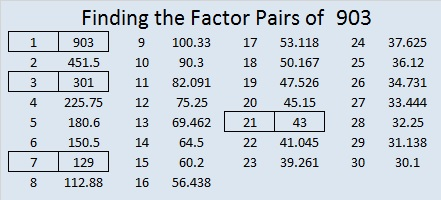# 903 Mystery Level

903 can be written as the sum of consecutive numbers seven different ways:

• 451 + 452 = 903; that’s 2 consecutive numbers.
• 300 + 301 + 302 = 903; that’s 3 consecutive numbers.
• 147 + 148 + 149 + 150 + 151 + 153 = 903; that’s 6 consecutive numbers.
• 126 + 127 + 128 + 129 + 130 + 131 + 132 = 903; that’s 7 consecutive numbers.
• 58 + 59 + 60 + 61 + 62 + 63 + 64 + 65 + 66 + 67 + 68 + 69 + 70 + 71 = 903; that’s fourteen consecutive numbers.
• 33 + 34 + 35 + 36 + 37 + 38 + 39 + 40 + 41 + 42 + 43 + 44 + 45 + 46 + 47 + 48 + 49 + 50 + 51 + 52 + 53 = 903; that’s 21 consecutive numbers.
• 1 + 2 + 3 + 4 + 5 + 6 + 7 + 8 + 9 + 10 + 11 + 12 + 13 + 14 + 15 + 16 + 17 + 18 + 19 + 20 + 21 + 22 + 23 + 24 + 25 + 26 + 27 + 28 + 29 + 30 + 31 + 32 + 33 + 34 + 35 + 36 + 37 + 38 + 39 + 40 + 41 + 42 = 903; that’s 42 consecutive numbers.

That last way means that 903 is the 42nd triangular number. It happened because (42 × 43)/2 = 903.Print the puzzles or type the solution on this excel file: 10-factors-897-904

• 903 is a composite number.
• Prime factorization: 903 = 3 × 7 × 43
• The exponents in the prime factorization are 1, 1, and 1. Adding one to each and multiplying we get (1 + 1)(1 + 1)(1 + 1) = 2 × 2 × 2 = 8. Therefore 903 has exactly 8 factors.
• Factors of 903: 1, 3, 7, 21, 43, 129, 301, 903
• Factor pairs: 903 = 1 × 903, 3 × 301, 7 × 129, or 21 × 43
• 903 has no square factors that allow its square root to be simplified. √903 ≈ 30.0499584.This site uses Akismet to reduce spam. Learn how your comment data is processed.